# Visually Explaining N1QL JOINs

### If you're using Couchbase, you likely know of N1QL. See how N1QL supports, or can mimic, seven classic SQL JOINs as well as some charts to show what you're JOINing.

· Database Zone · Tutorial
Save
19.22K Views

Couchbase's N1QL supports lookup joins, which allow only left-to-right JOINs, which means each qualified document from the left-hand side (LHS) of the JOIN operator is required to produce primary keys of documents on the right-hand side (RHS). These keys are subsequently nested-loop-joined to access qualified RHS documents. Couchbase Server version 4.1 and earlier only supported lookup joins.

But we can use lookup joins to implement [INNER JOIN] / [LEFT JOIN] / [RIGHT JOIN] / [OUTER JOIN] / [LEFT JOIN EXCLUDING INNER JOIN] / [RIGHT JOIN EXCLUDING INNER JOIN] / [OUTER JOIN EXCLUDING INNER JOIN].

I changed the Visual SQL JOINs examples to N1QL version, which:

1. Map table_A and table_B to a default bucket.

2. Add a "type" property with value “A” for table_A and value “B” for table_B into the document;

3. Change the primary key to ("type" || ":" || PK);

The N1QL examples would work equally across multiple buckets because N1QL can also JOIN across multiple buckets. I mapped both tables to the default bucket to make it easier for readers to run the examples in their own Couchbase environments.

``````INSERT INTO `default` (KEY, VALUE) VALUES ( "A:1",  {"type":"A","value":"FOX"}) RETURNING *;

INSERT INTO `default` (KEY, VALUE) VALUES ( "A:2",  {"type":"A","value":"COP"}) RETURNING *;

INSERT INTO `default` (KEY, VALUE) VALUES ( "A:3",  {"type":"A","value":"TAXI"}) RETURNING *;

INSERT INTO `default` (KEY, VALUE) VALUES ( "A:4",  {"type":"A","value":"LINCOLN"}) RETURNING *;

INSERT INTO `default` (KEY, VALUE) VALUES ( "A:5",  {"type":"A","value":"ARIZONA"}) RETURNING *;

INSERT INTO `default` (KEY, VALUE) VALUES ( "A:6",  {"type":"A","value":"WASHINGTON"}) RETURNING *;

INSERT INTO `default` (KEY, VALUE) VALUES ( "A:7",  {"type":"A","value":"DELL"}) RETURNING *;

INSERT INTO `default` (KEY, VALUE) VALUES ( "A:10", {"type":"A","value":"LUCENT"}) RETURNING *;

INSERT INTO `default` (KEY, VALUE) VALUES ( "B:1",  {"type":"B","value":"TROT"}) RETURNING *;

INSERT INTO `default` (KEY, VALUE) VALUES ( "B:2",  {"type":"B","value":"CAR"}) RETURNING *;

INSERT INTO `default` (KEY, VALUE) VALUES ( "B:3",  {"type":"B","value":"CAB"}) RETURNING *;

INSERT INTO `default` (KEY, VALUE) VALUES ( "B:6",  {"type":"B","value":"MONUMENT"}) RETURNING *;

INSERT INTO `default` (KEY, VALUE) VALUES ( "B:7",  {"type":"B","value":"PC"}) RETURNING *;

INSERT INTO `default` (KEY, VALUE) VALUES ( "B:8",  {"type":"B","value":"MICROSOFT"}) RETURNING *;

INSERT INTO `default` (KEY, VALUE) VALUES ( "B:9",  {"type":"B","value":"APPLE"}) RETURNING *;

INSERT INTO `default` (KEY, VALUE) VALUES ( "B:11", {"type":"B","value":"SCOTCH"}) RETURNING *;``````

## Inner JOINThis query will return all of the records in the left table (table A) that have a matching record in the right table (table B).

This JOIN, in SQL, is written as follows:

``````SELECT <select_list>

FROM Table_A A

INNER JOIN Table_B B

ON A.Key = B.Key``````

This JOIN, in N1QL, is written as follows:

``````SELECT <select_list>

FROM Table_A A

INNER JOIN Table_B B

ON KEYS <keys-clause(A)>``````

Note that keys-clause is required after each JOIN in N1QL. It specifies the primary keys for the second keyspace in the JOIN.

<keys-clause(A)> here means that it is a clause of constructing the primary key of RHS from the primary key of LHS.

For example, we can use a META() function to get the metadata for the document of Table_A. And we can use META(Table_A).id to get the primary key of the document of Table_A. And...

``ON KEYS ("B:" || (SPLIT(META(A).id,":")))``

... means that the matching relation is looks like as follows in SQL

``ON KEY  ("B:" || (SPLIT(META(A).id,":"))) = META(B).id``

So documents with the primary key “A:1” will match the document with the primary key “B:1”.

## Left JOINThis query will return all of the records in the left table (table A) regardless of whether any of those records have a match in the right table (table B). It will also return any matching records from the right table.

This JOIN, in SQL, is written as follows:

``````SELECT <select_list>

FROM Table_A A

LEFT JOIN Table_B B

ON A.Key = B.Key``````

This JOIN, in N1QL, is written as follows:

``````SELECT <select_list>

FROM Table_A A

LEFT JOIN Table_B B

ON KEYS <keys-clause(A)>``````

## Right JOIN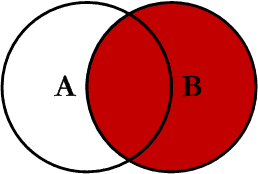This query will return all of the records in the right table (table B) regardless of whether any of those records have a match in the left table (table A). It will also return any matching records from the left table.

N1QL doesn’t support Right JOINs directly, but we can rewrite the query using a LEFT JOIN.

This JOIN, in SQL, is written as follows:

``````SELECT <select_list>

FROM Table_A A

RIGHT JOIN Table_B B

ON A.Key = B.Key``````

This JOIN, in N1QL, is written as follows by using a LEFT JOIN. Note that Table_B has been moved to the left-hand side in order to simulate a RIGHT JOIN.

``````SELECT <select_list>

FROM Table_B B

LEFT JOIN Table_A A

ON KEYS <keys-clause(B)>``````

## Full Outer JOIN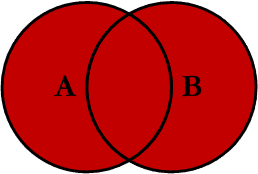This JOIN can also be referred to as a FULL OUTER JOIN or a FULL JOIN. This query will return all of the records from both tables, joining records from the left table (table A) that match records from the right table (table B).

N1QL does not support Full Outer JOINs directly, but we can rewrite the query using LEFT JOIN + LEFT  EXCLUDING JOIN or RIGHT JOIN + RIGHT  EXCLUDING JOIN.

This JOIN, in SQL, is written as follows:

``````SELECT <select_list>

FROM Table_A A

FULL OUTER JOIN Table_B B

ON A.Key = B.Key``````

This JOIN, in N1QL, is written as follows:

``````SELECT <select_list>

FROM Table_B B

LEFT JOIN Table_A A

ON KEYS <keys-clause(B)>

UNION ALL

SELECT <select_list>

FROM Table_A A

LEFT JOIN Table_B B

ON KEYS <keys-clause(A)>

WHERE META(B).id IS MISSING``````

## Left Excluding JOIN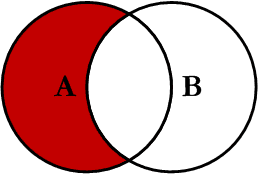This query will return all of the records in the left table (table A) that do not match any records in the right table (table B).

This JOIN, in SQL, is written as follows:

``````
SELECT <select_list>

FROM Table_A A

LEFT JOIN Table_B B

ON A.Key = B.Key

WHERE B.Key IS NULL``````

This JOIN, in N1QL, is written as follows:

``````SELECT <select_list>

FROM Table_A A

LEFT JOIN Table_B B

ON KEYS <keys-clause(A)>

WHERE META(B).id IS MISSING``````

## Right Excluding JOIN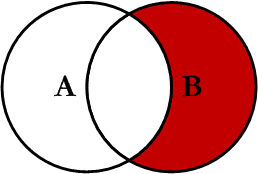This query will return all of the records in the right table (table B) that do not match any records in the left table (table A).

This JOIN, in SQL, is written as follows:

``````SELECT <select_list>

FROM Table_A A

RIGHT JOIN Table_B B

ON A.Key = B.Key

WHERE A.Key IS NULL``````

This JOIN, in N1QL, is written as follows by using a Left Excluding JOIN. Again, note that Table_B has been moved to the left-hand side to simulate a RIGHT JOIN.

``````SELECT <select_list>

FROM Table_B B

LEFT JOIN Table_A A

ON KEYS <keys-clause(B)>

WHERE META(B).id IS MISSING``````

## Outer Excluding JOINThis query will return all of the records in the left table (table A) and all of the records in the right table (table B) that do not match. I have yet to have a need for using this type of JOIN, but I use the other JOINs quite frequently. I will write about my application and uses in another article.

N1QL does not support Outer Excluding JOINs directly, but we can rewrite the query using LEFT EXCLUDING JOIN + RIGHT  EXCLUDING JOIN.

This JOIN, in SQL, is written as follows:

``````SELECT <select_list>

FROM Table_A A

FULL OUTER JOIN Table_B B

ON A.Key = B.Key

WHERE A.Key IS NULL OR B.Key IS NULL``````

This JOIN, in N1QL, is written as follows:

``````SELECT <select_list>

FROM Table_B B

LEFT JOIN Table_A A

ON KEYS <keys-clause(B)>

WHERE META(A).id IS MISSING

UNION ALL

SELECT <select_list>

FROM Table_A A

LEFT JOIN Table_B B

ON KEYS <keys-clause(A)>

WHERE META(B).id IS MISSING``````

## Examples

The results of the seven JOINs are shown below:

``````/* INNER JOIN */

SELECT SPLIT(META(A).id,":") AS A_PK, A.`value` AS A_Value,

B.`value` AS B_Value, SPLIT(META(B).id,":") AS B_PK

FROM default A

INNER JOIN default B

ON KEYS ("B:" || (SPLIT(META(A).id,":")))

WHERE A.type == "A"

/* "resultCount": 5 */

[

{

"A_PK": "1",

"A_Value": "FOX",

"B_PK": "1",

"B_Value": "TROT"

},

{

"A_PK": "2",

"A_Value": "COP",

"B_PK": "2",

"B_Value": "CAR"

},

{

"A_PK": "3",

"A_Value": "TAXI",

"B_PK": "3",

"B_Value": "CAB"

},

{

"A_PK": "6",

"A_Value": "WASHINGTON",

"B_PK": "6",

"B_Value": "MONUMENT"

},

{

"A_PK": "7",

"A_Value": "DELL",

"B_PK": "7",

"B_Value": "PC"

}

]``````

``````/* LEFT JOIN */

SELECT SPLIT(META(A).id,":") AS A_PK, A.`value` AS A_Value,

B.`value` AS B_Value, SPLIT(META(B).id,":") AS B_PK

FROM default A

LEFT JOIN default B

ON KEYS ("B:" || (SPLIT(META(A).id,":")))

WHERE A.type == "A"

/* "resultCount": 8 */

[

{

"A_PK": "1",

"A_Value": "FOX",

"B_PK": "1",

"B_Value": "TROT"

},

{

"A_PK": "10",

"A_Value": "LUCENT"

},

{

"A_PK": "2",

"A_Value": "COP",

"B_PK": "2",

"B_Value": "CAR"

},

{

"A_PK": "3",

"A_Value": "TAXI",

"B_PK": "3",

"B_Value": "CAB"

},

{

"A_PK": "4",

"A_Value": "LINCOLN"

},

{

"A_PK": "5",

"A_Value": "ARIZONA"

},

{

"A_PK": "6",

"A_Value": "WASHINGTON",

"B_PK": "6",

"B_Value": "MONUMENT"

},

{

"A_PK": "7",

"A_Value": "DELL",

"B_PK": "7",

"B_Value": "PC"

}

]

``````

``````/* RIGHT JOIN */

SELECT SPLIT(META(A).id,":") AS A_PK, A.`value` AS A_Value,

B.`value` AS B_Value, SPLIT(META(B).id,":") AS B_PK

FROM default B

LEFT JOIN default A

ON KEYS ("A:" || (SPLIT(META(B).id,":")))

WHERE B.type == "B"

/* "resultCount": 8 */

[

{

"A_PK": "1",

"A_Value": "FOX",

"B_PK": "1",

"B_Value": "TROT"

},

{

"B_PK": "11",

"B_Value": "SCOTCH"

},

{

"A_PK": "2",

"A_Value": "COP",

"B_PK": "2",

"B_Value": "CAR"

},

{

"A_PK": "3",

"A_Value": "TAXI",

"B_PK": "3",

"B_Value": "CAB"

},

{

"A_PK": "6",

"A_Value": "WASHINGTON",

"B_PK": "6",

"B_Value": "MONUMENT"

},

{

"A_PK": "7",

"A_Value": "DELL",

"B_PK": "7",

"B_Value": "PC"

},

{

"B_PK": "8",

"B_Value": "MICROSOFT"

},

{

"B_PK": "9",

"B_Value": "APPLE"

}

]``````

``````/* OUTER JOIN */

SELECT SPLIT(META(A).id,":") AS A_PK, A.`value` AS A_Value,

B.`value` AS B_Value, SPLIT(META(B).id,":") AS B_PK

FROM default B

LEFT JOIN default A

ON KEYS ("A:" || (SPLIT(META(B).id,":")))

WHERE B.type == "B"

UNION ALL

SELECT SPLIT(META(A).id,":") AS A_PK, A.`value` AS A_Value,

B.`value` AS B_Value, SPLIT(META(B).id,":") AS B_PK

FROM default A

LEFT JOIN default B

ON KEYS ("B:" || (SPLIT(META(A).id,":")))

WHERE A.type == "A"

AND META(B).id IS MISSING

/* "resultCount": 11 */

[

{

"A_PK": "10",

"A_Value": "LUCENT"

},

{

"A_PK": "4",

"A_Value": "LINCOLN"

},

{

"A_PK": "5",

"A_Value": "ARIZONA"

},

{

"A_PK": "1",

"A_Value": "FOX",

"B_PK": "1",

"B_Value": "TROT"

},

{

"B_PK": "11",

"B_Value": "SCOTCH"

},

{

"A_PK": "2",

"A_Value": "COP",

"B_PK": "2",

"B_Value": "CAR"

},

{

"A_PK": "3",

"A_Value": "TAXI",

"B_PK": "3",

"B_Value": "CAB"

},

{

"A_PK": "6",

"A_Value": "WASHINGTON",

"B_PK": "6",

"B_Value": "MONUMENT"

},

{

"A_PK": "7",

"A_Value": "DELL",

"B_PK": "7",

"B_Value": "PC"

},

{

"B_PK": "8",

"B_Value": "MICROSOFT"

},

{

"B_PK": "9",

"B_Value": "APPLE"

}

]``````

``````/* LEFT EXCLUDING JOIN */

SELECT SPLIT(META(A).id,":") AS A_PK, A.`value` AS A_Value,

B.`value` AS B_Value, SPLIT(META(B).id,":") AS B_PK

FROM default A

LEFT JOIN default B

ON KEYS ("B:" || (SPLIT(META(A).id,":")))

WHERE A.type == "A"

AND META(B).id IS MISSING

/* "resultCount": 3 */

[

{

"A_PK": "10",

"A_Value": "LUCENT"

},

{

"A_PK": "4",

"A_Value": "LINCOLN"

},

{

"A_PK": "5",

"A_Value": "ARIZONA"

}

]``````

``````/* RIGHT EXCLUDING JOIN */

SELECT SPLIT(META(A).id,":") AS A_PK, A.`value` AS A_Value,

B.`value` AS B_Value, SPLIT(META(B).id,":") AS B_PK

FROM default B

LEFT JOIN default A

ON KEYS ("A:" || (SPLIT(META(B).id,":")))

WHERE B.type == "B"

AND META(A).id IS MISSING

/* "resultCount": 3 */

[

{

"B_PK": "11",

"B_Value": "SCOTCH"

},

{

"B_PK": "8",

"B_Value": "MICROSOFT"

},

{

"B_PK": "9",

"B_Value": "APPLE"

}

]``````

``````/* OUTER EXCLUDING JOIN */

SELECT SPLIT(META(A).id,":") AS A_PK, A.`value` AS A_Value,

B.`value` AS B_Value, SPLIT(META(B).id,":") AS B_PK

FROM default B

LEFT JOIN default A

ON KEYS ("A:" || (SPLIT(META(B).id,":")))

WHERE B.type == "B"

AND META(A).id IS MISSING

UNION ALL

SELECT SPLIT(META(A).id,":") AS A_PK, A.`value` AS A_Value,

B.`value` AS B_Value, SPLIT(META(B).id,":") AS B_PK

FROM default A

LEFT JOIN default B

ON KEYS ("B:" || (SPLIT(META(A).id,":")))

WHERE A.type == "A"

AND META(B).id IS MISSING

/* "resultCount": 6 */

[

{

"B_PK": "11",

"B_Value": "SCOTCH"

},

{

"B_PK": "8",

"B_Value": "MICROSOFT"

},

{

"B_PK": "9",

"B_Value": "APPLE"

},

{

"A_PK": "10",

"A_Value": "LUCENT"

},

{

"A_PK": "4",

"A_Value": "LINCOLN"

},

{

"A_PK": "5",

"A_Value": "ARIZONA"

}

]``````

## N1QL supports most of our JOINs directly. It does not support RIGHT JOIN/OUTER JOIN/OUTER EXCLUDING JOINs, but we can rewrite the query to get all kinds of JOINs.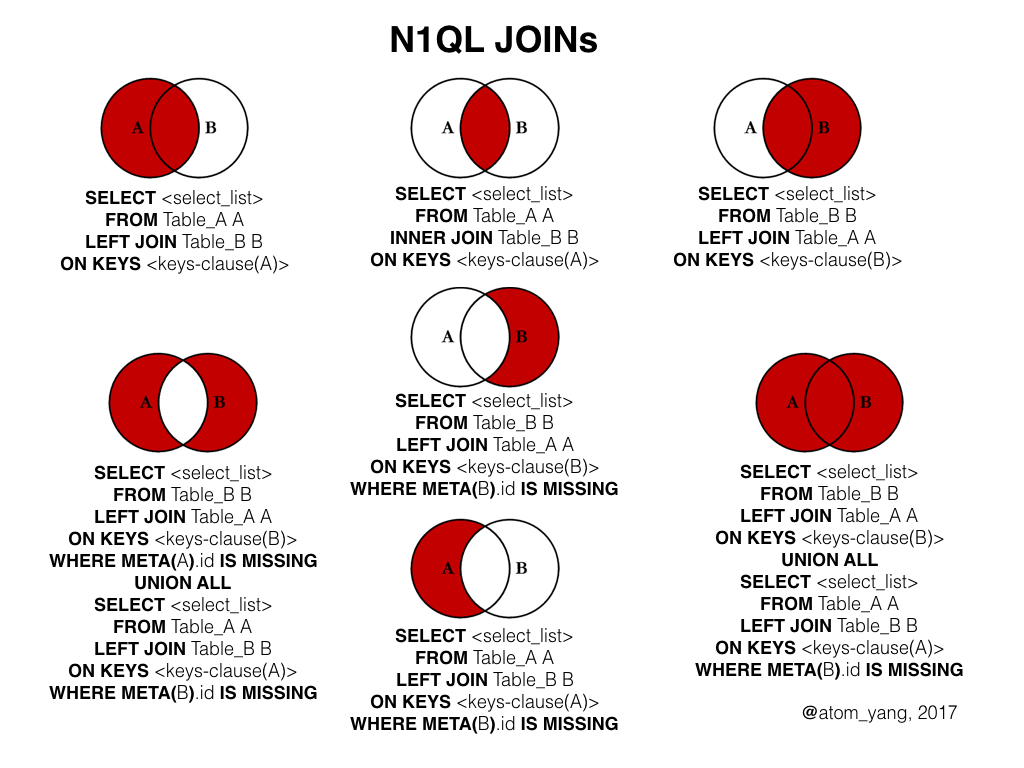Topics:
n1ql, sql, join, database, tutorial

Opinions expressed by DZone contributors are their own.